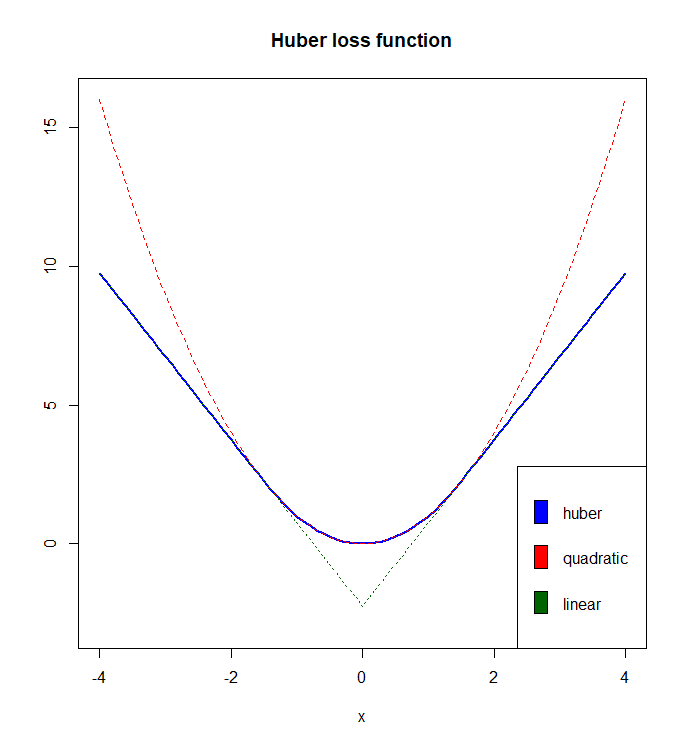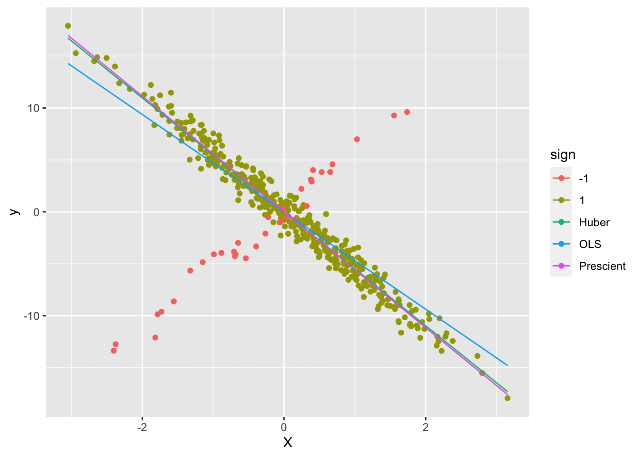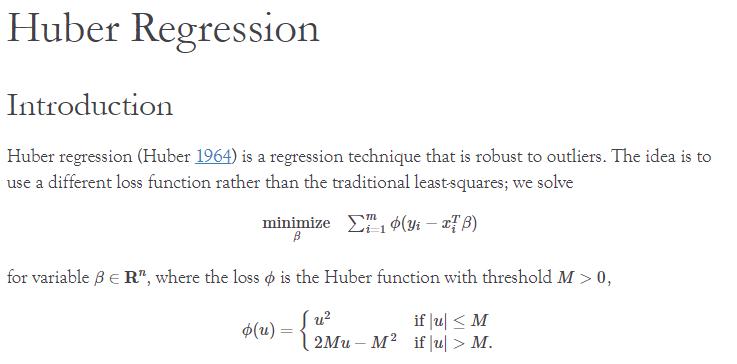## Friday, September 10, 2021

### Huber regression: different formulationsPeter Huber, Swiss statistician

Traditionally, regression, well-known and widely used, is based on a least-squares objective: we have quadratic costs. A disadvantage of this approach is that large deviations (say due to outliers) get a very large penalty. As a result, these outliers can affect the results dramatically.

One alternative regression method that tries to remedy this is L1- or LAD-regression (LAD=Least Absolute Deviation) . Here I want to discuss a method that is somewhere in the middle between L1 regression and least-squares: Huber or M-Regression .  Huber regression uses a loss function that is quadratic for small deviations and linear for large ones. It is defined in a piecewise fashion. The regression can look like the following non-linear optimization problem:

NLP Model
\begin{align} \min_{\color{darkred}\beta,\color{darkred}\varepsilon} &\sum_i \rho(\color{darkred}\varepsilon_i) \\ &\color{darkblue}y_i = \sum_j \color{darkblue}x_{i,j}\color{darkred}\beta_j +\color{darkred}\varepsilon_i \end{align} where $\rho(\color{darkred}\varepsilon_i) = \begin{cases}\color{darkred}\varepsilon^2_i & \text{if |\color{darkred}\varepsilon_i | \le \color{darkblue}k} \\ 2\color{darkblue}k |\color{darkred}\varepsilon_i |-\color{darkblue}k^2 & \text{otherwise}\end{cases}$The piecewise linear and quadratic Huber loss function $$\rho(x)$$

The picture shows the Huber loss function $$\rho(x)$$  with $$\color{darkblue}k = 1.5$$. We see that for $$x \in [-1.5, 1.5]$$ we have a quadratic behavior (following the red line), while for deviations larger than 1.5 we use linear penalties (using the green line). The Huber function is continuous and differentiable (but not twice continuously differentiable).

Huber regression requires you to provide the parameter $$\color{darkblue}k$$. This is sometimes called the robustification parameter. There are methods that will try to determine $$\color{darkblue}k$$ automatically. Such methods are called adaptive or tuning-free. I assume we provide $$\color{darkblue}k$$ exogenously.

Below I will briefly describe four different mathematical programming formulations: an NLP, an LCP, and two different QP models.

#### Solve as NLP

We can directly solve this as an NLP (non-linear programming problem). In GAMS this can look like:

 $ontext Huber M regression by NLP erwin@amsterdamoptimization.com$offtext set i 'number of cases' /i1*i40/; set j 'coefficient to estimate' /'constant','coeff1'/; include expdata.inc parameter y(i); y(i) = data(i,'expenditure'); parameter X(i,j); X(i,'constant') = 1; X(i,'coeff1') = data(i,'income'); scalar k /1.5/; variables loss 'objective' e(i) 'deviations' beta(j) 'parameters to estimate' ; equations obj fit(i) ; obj.. loss =e= sum(i, ifthen(abs(e(i))<=k, sqr(e(i)), 2*k*abs(e(i))-sqr(k))); fit(i).. y(i) =e= sum(j, X(i,j)*beta(j)) + e(i); model m /all/; solve m using dnlp minimizing loss; display beta.l; Usually, absolute values are very dangerous. In GAMS, to warn you against this, you need to solve this NLP model as a DNLP model. In this case, the danger is limited, as the objective is convex, continuous and differentiable. The second derivatives are not continuous, however. This is the only version that is really intuitive. The other formulations below do work just fine, but I find them not at all obvious. If there is an intuitive interpretation, I would love to hear it. #### Solve as LCP In [3,4] it is proposed to form the first-order optimality conditions of this problem and solve the resulting LCP (linear complementarity problem). The LCP looks like: LCP Model \begin{align} \color{darkred}\lambda_i +\color{darkblue}y_i - \sum_j\color{darkblue}X_{i,j} \color{darkred}\beta_j &= \color{darkred}s^+_i - \color{darkred}s^-_i && \forall i \\ \sum_i \color{darkblue}X_{i,j}\color{darkred}\lambda_i & =0&& \forall j \\ \color{darkred}\lambda_i + \color{darkblue}k \ge 0 &\perp \color{darkred}s^+_i \ge 0 && \forall i \\ -\color{darkred}\lambda_i + \color{darkblue}k \ge 0 &\perp \color{darkred}s^-_i \ge 0 && \forall i \\ \color{darkred}s^+_i,\color{darkred}s^-_i &\ge 0 \end{align} Here $$\perp$$ indicates complementarity between a constraint and a variable. Note that this model is a system of equations: there is no objective In GAMS the equations look like: ontext    Huber M regression by LCP    erwin@amsterdamoptimization.com $offtext set i 'number of cases' /i1*i40/; set j 'coefficient to estimate' /'constant','coeff1'/;$include expdata.inc parameter y(i); y(i) = data(i,'expenditure'); parameter X(i,j); X(i,'constant') = 1; X(i,'coeff1') = data(i,'income'); scalar k /1.5/; variables    lambda(i)  'lagrange multipliers'    beta(j)    'parameters to estimate'    sp(i)      'slacks'    sm(i)      'slacks' ; positive variables sp,sm; equations    e1(i)    e2(j)    compl1(i)    compl2(i) ; e1(i).. lambda(i)  + y(i) - sum(j, X(i,j)*beta(j)) =e=  sp(i) - sm(i); e2(j).. sum(i, X(i,j)*lambda(i)) =e= 0; compl1(i).. lambda(i) + k =g= 0; compl2(i).. -lambda(i) + k =g= 0; model m /e1,e2,compl1.sp,compl2.sm/; solve m using mcp; display beta.l;

GAMS does not have an LCP solver so instead, we use a more general MCP (Mixed Complementarity) solver.

We know we can solve LCPs in different ways . A QP formulation is the next candidate.

#### Solve as QP

In , the following convex QP formulation is proposed:

QP Model
\begin{align}\min\> & 0.5 \sum_i \color{darkred}\varepsilon_i^2 + \color{darkblue}k \sum_i \color{darkred}t_i \\ & -\color{darkred}t_i\le \sum_j \color{darkblue}X_{i,j} \color{darkred}\beta_j - \color{darkblue}y_i + \color{darkred}\varepsilon_i \le \color{darkred}t_i && \forall i\\ & \color{darkred}t_i\ge 0\end{align}

This looks a bit like normal regression with a regularization term.

This formulation can be fed into a standard QP solver.

 variables    loss     'objective'    e(i)     'deviations'    beta(j)  'parameters to estimate'    t(i)     'absolute value' ; positive variable t; equations    obj    bound1(i)    bound2(i) ; obj.. loss =e= 0.5*sum(i,sqr(e(i))) + k*sum(i,t(i)); bound1(i).. -t(i) =l= sum(j, X(i,j)*beta(j)) - y(i) + e(i); bound2(i).. t(i) =g= sum(j, X(i,j)*beta(j)) - y(i) + e(i); model m /all/; solve m using qcp minimizing loss; display beta.l;

Notes:

• We can make $$\color{darkred}t_i$$ a free variable. The model will automatically make it non-negative. In my experience using a positive variable can help the solver a bit.
• We should get rid of the duplicate expression by introducing a new variable and a new equality constraint.
• A variant of this model  is based on variable splitting: \begin{align}\min\> & 0.5 \sum_i \color{darkred}\varepsilon_i^2 + \color{darkblue}k \sum_i \left(\color{darkred}s^+_i+\color{darkred}s^-_i\right) \\ & \color{darkred}\varepsilon_i- \sum_j \color{darkblue}X_{i,j} \color{darkred}\beta_j - \color{darkblue}y_i +\color{darkred}s^+_i - \color{darkred}s^-_i = 0 && \forall i\\ & \color{darkred}s^+_i,\color{darkred}s^-_i\ge 0\end{align}

#### Solve as dual QP

A dual formulation  is:

Dual QP Model
\begin{align} \min & \frac{\color{darkblue}k}{2}\sum_i \color{darkred}u_i^2 + \sum_i \color{darkblue}y_i \color{darkred}u_i \\ &\sum_i \color{darkblue}X_{i,j}\color{darkred}u_i = 0 \perp \color{darkred}\beta_j && \forall j \\ & \color{darkred}u_i \in [-1,1]\end{align}

The GAMS code can look like:

 variables    loss     'objective'    u(i)     'duals' ; equations    obj    edual(j) ; obj.. loss =e= (k/2)*sum(i,sqr(u(i))) + sum(i, y(i)*u(i)); edual(j).. sum(i, X(i,j)*u(i)) =e= 0; u.lo(i) = -1; u.up(i) = 1; model m /all/; solve m using qcp minimizing loss; display edual.m;

Note that we can retrieve the estimates for $$\color{darkred}\beta$$ by inspecting the duals (marginals) of equation edual.

#### Other tools

CVXPY and CVXR have a built-in function huber(). This will allow you to do Huber regression from CVXPY and CVXR. In  a great example is used to illustrate Huber regression:Example from 

The problem description contains a small error:The function $$\phi(u)$$ is missing an $$|.|$$

The function $$\phi(u)$$ should use $$|u|$$ instead of $$u$$.

R has the function rlm from the MASS package.

#### Conclusion

The Huber regression problem for robust regression can be solved in different ways.  I have presented here four different implementations (NLP, LCP, and primal and dual QP). The quadratic formulations are likely the best candidates for large problems.

It is not so obvious that we can formulate compact standard QP models for this problem. This is interesting not only for robust regression problems but also in other cases where we want to add a penalty term that is between linear and quadratic.

#### References

1. Linear Programming and L1 Regression, https://yetanothermathprogrammingconsultant.blogspot.com/2017/11/lp-and-lad-regression.html
2. Huber loss, https://en.wikipedia.org/wiki/Huber_loss
3. Michael Gertz and Stephen Wright, Object-oriented Software for Quadratic Programming, Tech. Report ANL/MCS-P891-1000, Argonne National Laboratory, Mathematics and Computer Science Division, 2001.
4. Stephen J. Wright, On reduced convex QP formulations of monotone LCPs, Mathematical Programming 90 (2001), 459–473.
5. Solving linear complementarity problems without an LCP solver, https://yetanothermathprogrammingconsultant.blogspot.com/2021/05/solving-linear-complementarity-problems.html
6. Olvi L. Mangasarian and David R. Musicant, Robust Linear and Support Vector Regression, IEEE transactions on pattern analysis and machine intelligence, vol. 22, no 9, 2000.
7. Huber Regression, https://cvxr.rbind.io/cvxr_examples/cvxr_huber-regression/